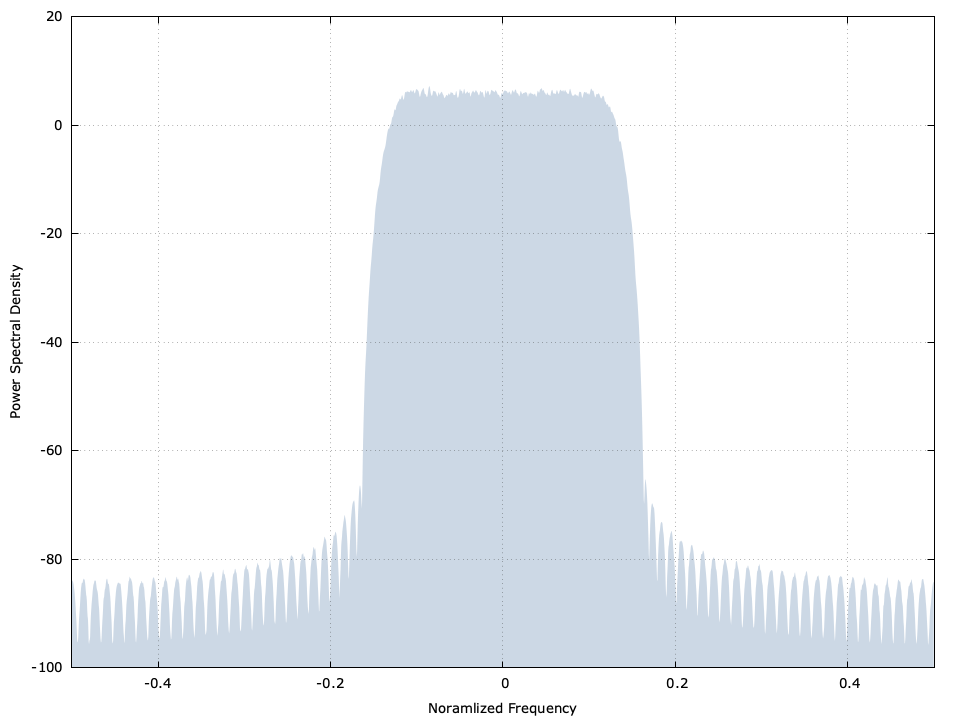# Symbol streamer (symstream)

The symstream family of objects generate a stream of linearly-modulated symbols interpolated with an appropriate pulse-shaping filter. The resulting data samples are stored in a buffer provided by the user. This object is intended for generating a signal source appropriate for testing synchronization techniques such as carrier recovery , symbol timing recovery , or estimating power spectral density .Figure [symstream-example]. symstreamcf example

## Example

The following listing creates the data in Figure [symstream-example] at the top of this page.


#include <stdio.h>
#include <stdlib.h>
#include <math.h>
#include <liquid/liquid.h>

int main()
{
// create stream generator
int          ftype   = LIQUID_FIRFILT_ARKAISER;
unsigned int k       =     4;
unsigned int m       =     9;
float        beta    = 0.30f;
int          ms      = LIQUID_MODEM_QPSK;
symstreamcf  gen     = symstreamcf_create_linear(ftype,k,m,beta,ms);

unsigned int buf_len = 1024;
float complex buf[buf_len];

// generate 50 buffers worth of samples
unsigned int i;
for (i=0; i<50; i++) {
// write samples to buffer
symstreamcf_write_samples(gen, buf, buf_len);

// do something with samples here
}

symstreamcf_destroy(gen);
return 0;
}